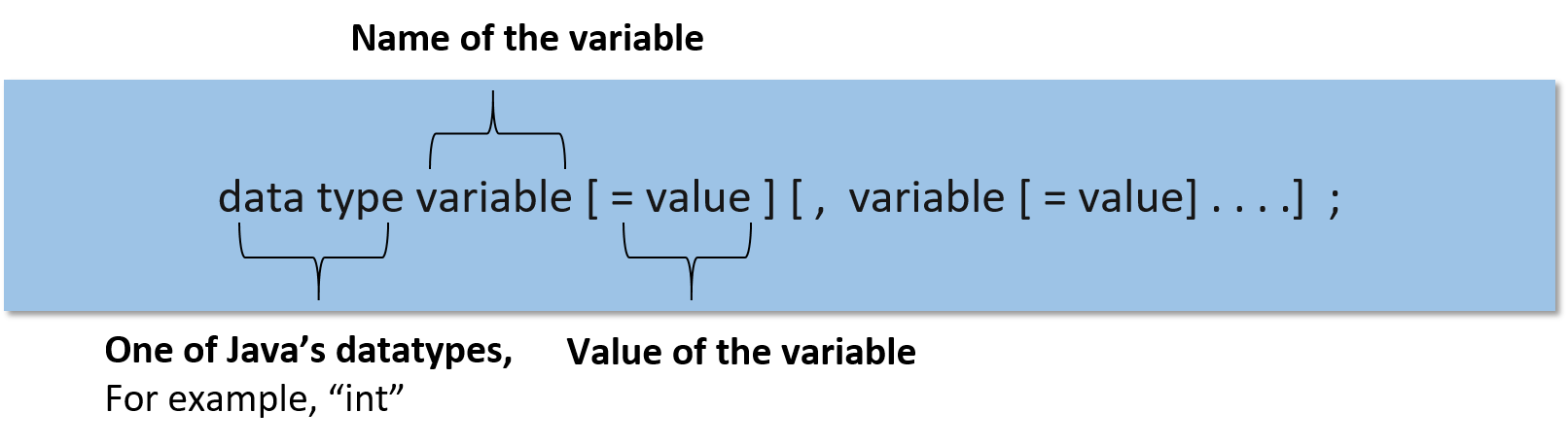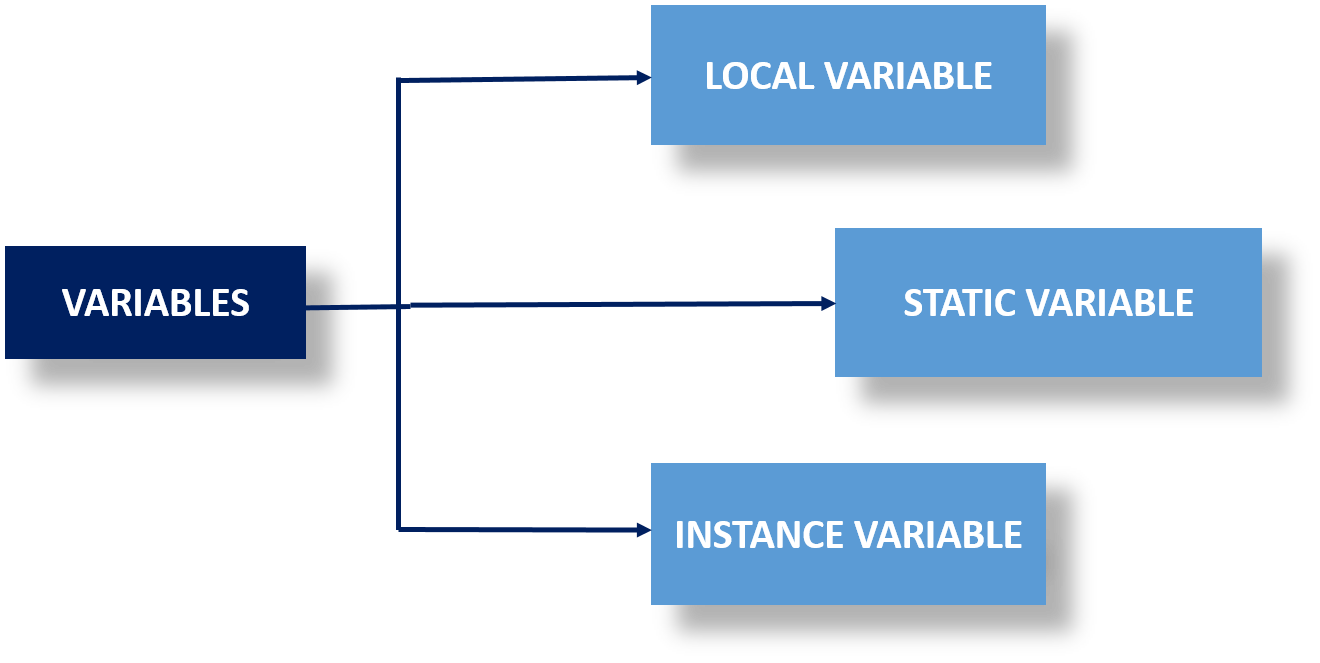# Variables in Java: Local, Instance and Static Variables

22 Sep 2023
Beginner
1.5K ViewsLearn via Video Course & by Doing Hands-on Labs

## Variables in Java: An Overview

Variables in Java serve as containers for storing data, allowing programmers to manipulate and work with information efficiently within their programs. To gain expertise in Java and harness its power for various applications, java Online Training is a convenient option. If you're interested in learning more about Java, variables in Java, and variables in Java example you can check out our Java tutorial for beginners!

## What is a Variable in Java?

Variables work as a container to store the values of data. It saves the data while the language is executing a program. Every Variable has a data type that understands and designates the quantity and type of the value that can be stored by the container. It is a memory location that memorizes the location of the data. Knowing about variables in Java is one of the most important reasons to learn about Java.

## Variable Declaration

There are various types of declaration that happen by using Java variables such as

• Strings- which stores text, Such as "Hello world".
• Int- it stores any integer, specifically any whole number without decimal.
• Float- stores "floating point" numbers that include decimal values
• Char- it stores characters such as 'x', and 'y'. It is denoted by single quotes
• Boolean- store values if true or false state.
To declare the variable programmers, need to focus on two things, are
1. datatype: it denotes the types of Data that are going to be stored
2. data_name: a particular name of that variable

#### Example

``````class Example
{
public static void main ( String[] args )
{
long payAmount = 184; //the declaration of the variable

System.out.println("The variable contains: " + payAmount );
}
}
``````
This program is an example of using the variable "payAmount".

#### Output

``````Long payAmount = 184;
``````

## Variable Initialization

For initializing the variable there are 3 major components, are
• datatype: it defines what type of data is going to be stored in a variable
• variable_name: Particular name of the variable
• value: initially stored value in the variable## Types of Variables in Java

There are three types of variables in Java:### Local variables

• It is created when a block is entered in the storage and then it calls and destroys the block just after exiting from the function.
• It is important to initialize a local variable before using it.

#### Example

``````import java.io.*;

class DNT
{
public static void main(String[] args)
{
int var = 89; // Declared a Local Variable

// This variable is local to this main method only

System.out.println("Local Variable: " + var);
}
}``````

This Java example illustrates the concept of local variables with a scope that is only the main method by declaring and initializing a local variable "var" with the value 89.

#### Output:

``````Local Variable: 89
``````

### Instance Variables

• An instance variable is created when an object class is generated and it gets destroyed when the same object class is destroyed
• Programmers can use access specifiers for instance variables
• It is not mandatory to initialize instance variables

#### Example

``````import java.io.*;
class DNT
{
public String student; // Declared Instance Variable
public DNT()
{ // Default Constructor
this.student= "Urmi Bose"; // initializing Instance Variable
}
//Main Method
public static void main(String[] args)
{
// Object Creation
DNT name = new DNT();
System.out.println("Student name is: " + name.student);
}
}``````

In this Java example, the class "DNT" contains a declaration for an instance variable named "student" that is initialized in the default constructor to demonstrate the use of instance variables. An object of the class is then constructed to allow access to and printing of the student's name.

#### Output

``````Student name is : Urmi Bose
``````

### Static variables

• Static variables are declared as instance variables.
• It is created to start the execution of the program and then destroy it automatically after the execution ends.
• It uses static keywords for the declaration of the static variable within a class

#### Example

``````import java.io.*;
class DNT
{
public static String student= "Urmi Bose"; //Declared static variable

public static void main (String[] args) {

//student variable can be accessed without object creation
System.out.println("Student Name is : "+DNT.student);
}
}``````

This Java example prints the value "Urmi Bose" from a static variable named "student" that is declared inside the class "DNT," enabling access by class name simply without creating an object.

#### Output:

``Student Name is: Urmi Bose``

## FAQs

### 1. What are the variables in Java?

In Java, variables serve as storage containers for data types like numbers, text, and objects.

### 2. What are the 3 types of variables in Java?

Local, instance and static variables are the three different categories of variables in Java.

### 3. What is a variable declaration in Java?

The data type and variable name must be specified when declaring a variable in Java.

### 4. What is variable initialization in Java?

Assigning an initial value to a declared variable in Java usually takes place on the same line of code or later.
##### Summary
This article covers the definition of the variable, variable declaration, variables in Java, variables in Java example, and variable initialization. Additionally, it provides a comprehensive understanding of different types of variables and, makes it a valuable resource for Java Certification preparation.
Share Article
Similar Articles